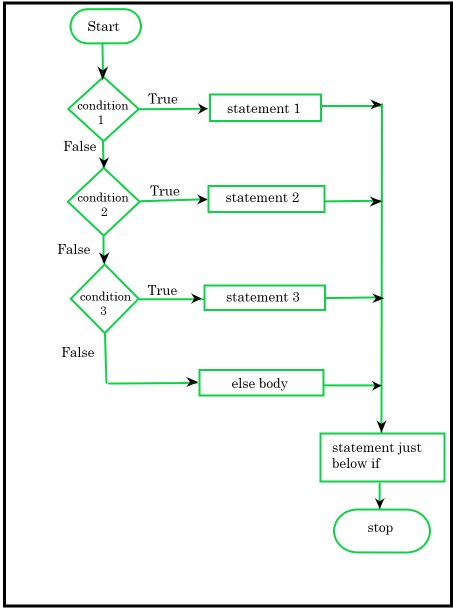Swift – If-else-if Statement

• Last Updated : 06 Dec, 2021

In Swift, the if-else if-else condition is used to execute a block of code among multiple options. It gives a choice between more than two alternative conditions as soon as one of the conditions is true, the statement associated with that if is executed. If all conditions are false or not satisfied then the final else statement will be executed in the program.

Syntax:

if (condition1)

{

// Block of code

// Statement 1

}

else if (condition2)

{

// block of code

// statement 2

}

.

.

.

else

{

// block of code

}

Here, If condition1 is true, then statement 1 is executed. If condition1 is false, then it will go to else if statement and evaluate condition 2. If condition 2 is true, statement 2 will be executed. If condition 2 is false, then it will go to else if statement and evaluate condition 3 likewise so on. If neither condition is true it will move to the else part and then the final else statement will be executed.

Flowchart:Example 1:

Swift

 // Swift program illustrate the use// of if-else-if statementimport Swiftlet number = 85  if (number >= 90){          // Print statement    print("Grade A")}  else if (number >= 75) {          // Print statement    print("Grade B")}  else {          // Print statement    print("Grade C")}

Output:

Explanation: In the above example, first we create a variable named “number”. Now using the if-else-if statement we check if the number is greater than or equal to 90, assigning grade A. Or if the number is greater than or equal to 75, assign grade B. Or if the number is greater than or equal to 60, assign grade C. So here the output will be Grade B because number = 85 which satisfies the condition “number is greater than or equal to 75”.

Example 2:

Swift

 // Swift program illustrate the use of// if-else-if statement import Swift  let number = 20  // Condition 1if (number == 10){          // Print statement    print("Number is 10")}  // Condition 2else if (number == 15){          // Print statement    print("Number is 15")}  // Condition 3else if (number == 20){          // Print statement    print("Number is 20")}else{          // Print statement    print("Number is not present")}

Output:

Number is 20

Explanation: In the above example, we created a variable that holds an expression and we have three condition expressions:

• if (number == 10) : checks if number equal to 10
• else if (number == 15) : checks if number is equal to 15.
• else if (number == 20) : checks if number is equal to 20.

Here, both conditions1 and condition2 are is false. Hence the statement will move to condition3(condition3 is true) and statement 3 is executed.

My Personal Notes arrow_drop_up